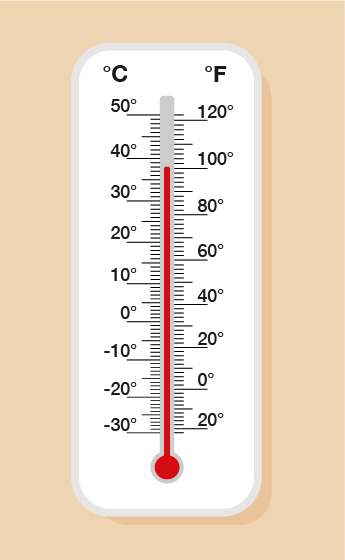Everyday maths 2 (Wales)

Start this free course now. Just create an account and sign in. Enrol and complete the course for a free statement of participation or digital badge if available.

Free course

# 5.3 Using conversion scales

Earlier on in the session you looked at converting between units of measure in different systems by carrying out calculations.

Many measuring instruments (e.g. thermometers, rulers, measuring jugs) have scales which show two or more different units of measure. This means that there may be times where you can compare the scales on the measuring instrument to make a conversion rather than carry out a calculation.

Look at the following example.Figure 32 Example – Reading a thermometer

The thermometer above has a scale down the left-hand side which shows degrees Celsius (°C) and a scale on the right-hand side which shows degrees Fahrenheit (°F). This means that you can take a reading on this thermometer in both units of measure, depending on which is needed or which you are more familiar with. It can also help you to look up conversions between units.

You need to be careful with each scale, though – as they are showing different units, they are marked differently and go up in different steps.

On this thermometer, the degrees Celsius scale is going up in steps of 1°C, so the temperature shown is 38°C. If you want to take the reading in degrees Fahrenheit, you can see that it is 100°F (the scale is going up in steps of 2°F). It can be difficult to get a precise comparison between units, but using this thermometer, we can say that 38°C is roughly 100°F.

Now have a go at the following activity.

## Activity 12: Using conversion scales

Look at the weighing scales below and answer the questions that follow.

1. What is the reading shown by the arrow in grams?

2. How many ounces (oz) is 200 g, to the nearest whole oz?

3. Roughly how many grams is 1 oz, to the nearest 10 g?

4. I see a recipe which states that I need 6 oz of flour. Roughly, how many grams of flour is this?

Grams (g) are shown on the outside of this scale and ounces (oz) are shown on the inside.

1. The arrow is pointing to 70 g (the scale is going up in steps of 5).

2. You need to look on the outside of the scale to find 200 g and then look on the inside to see how many whole ounces it is nearest to.

The nearest whole ounce is 7 oz.

3. Find 1 oz on the inside of the scale. Now look on the outside to take this reading in grams. The nearest marker is 30 grams (the grams scale goes up in steps of 5) so 1 oz is approximately 30 g.

4. Look on the inside of the scale for 6 oz. Then take the equivalent gram reading from the outside of the scale. 6 oz is approximately 170 g.

You have now learned all you need to know about units of measures! If you feel unsure on any part of this section, feel free to refer back to the examples or activities again to ensure you feel secure in all areas. All that remains of this section is the end of session quiz. Good luck!

## Summary

In this section you have learned to read:

• measuring scales using different intervals
• scales on different measuring instruments
• conversion scales.
FSM_2_CYMRU#### Find out more

*Eligibility rules apply for financial support.